# 1-理论基础

## 1.1-滤波器基本类型

• 低通滤波器（Low-Pass Filter，LPF）
• 高通滤波器（High-Pass Filter，HPF）
• 带通滤波器（Band-Pass Filter，BPF）
• 带阻滤波器（Band-Stop Filter，BSF）
• 全通滤波器（All-Pass Filter，APF）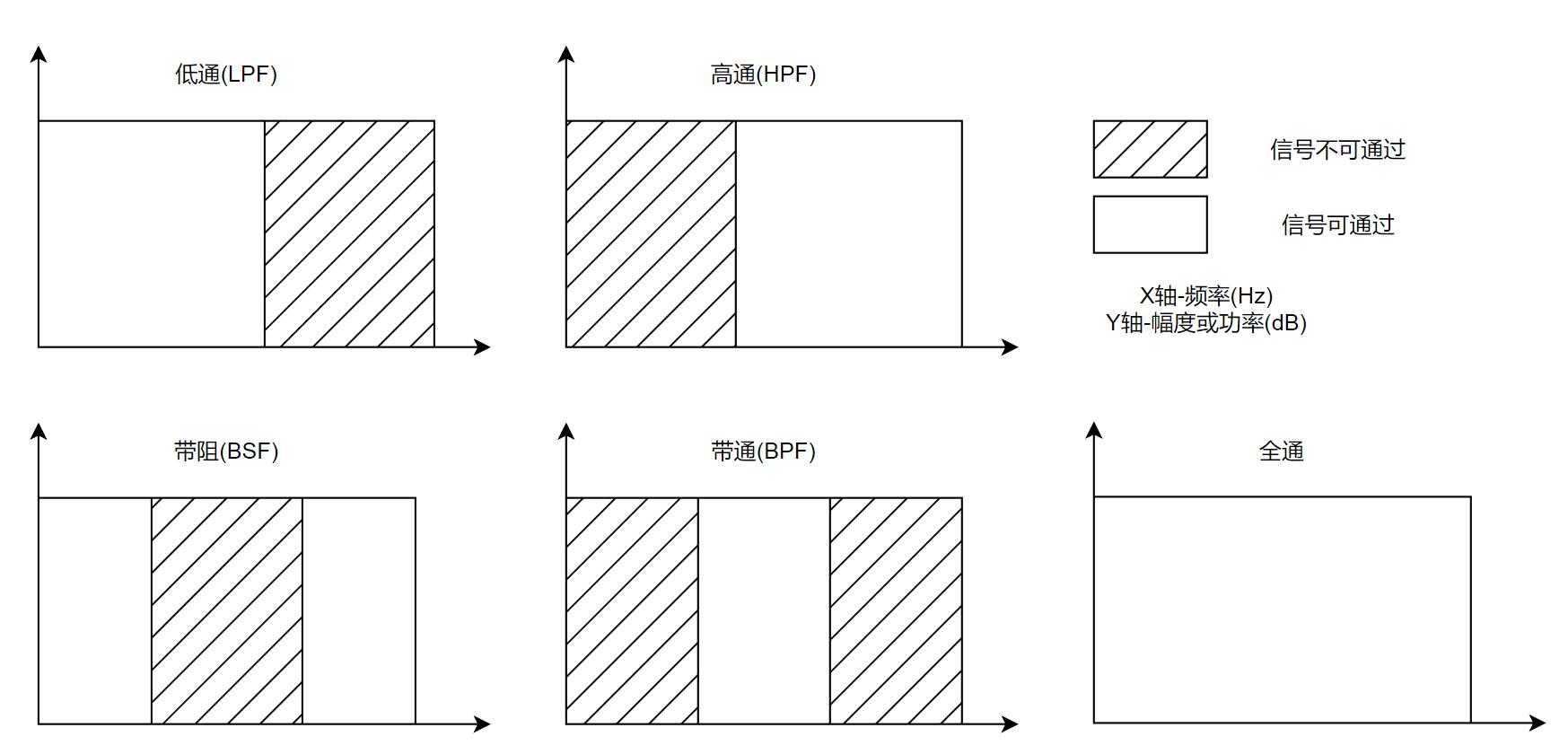• 巴特沃斯（Butterworth）
• 切比雪夫（Chebyshev）(有Ⅰ型和Ⅱ型)
• 贝塞尔（Bessel）
• 椭圆/考尔（Elliptic，或Cauer）

## 1.4-归一化(Normalize)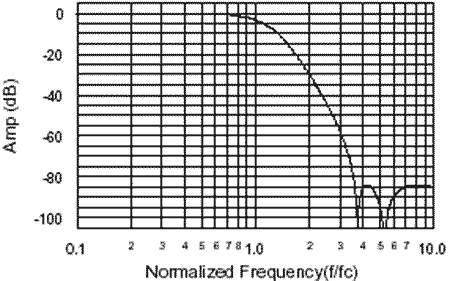# 2-滤波器重要参数

## 2.0-衰减/插损/抑制-术语辨析

• 衰减(Attenuation):某频点处,输出信号相对于原值衰减的比例，单位有时是数值，有时是dB，适用于所有描述滤波器的语境
• 插损(插入损耗,Insertion Loss,简写IL):定义为某频率处的衰减值，一般单位是dB，该词多用于射频系统中
• 抑制(Suppresion):一般用于描述滤波器在 阻带 某频率处的衰减大小。(通带就不能说"抑制"了…)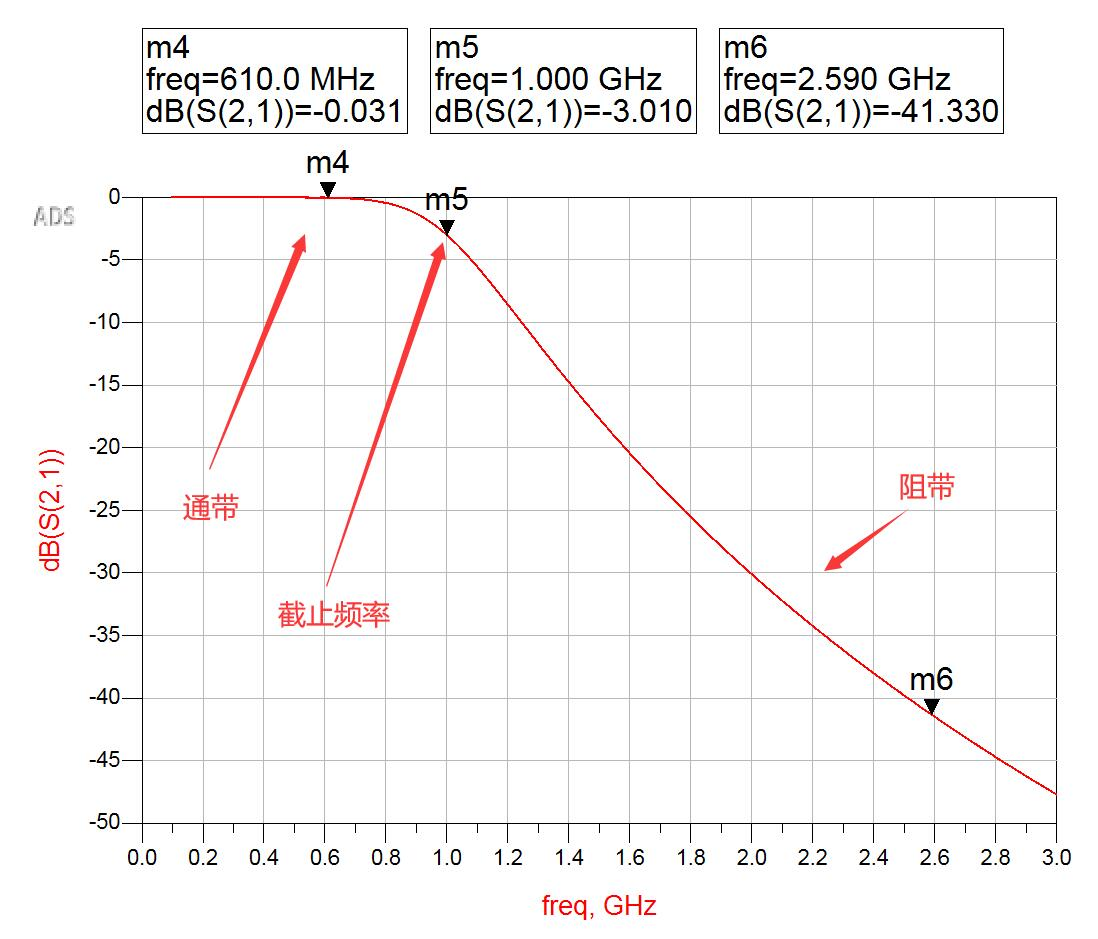## 2.1-幅度特性-通带/阻带的衰减特性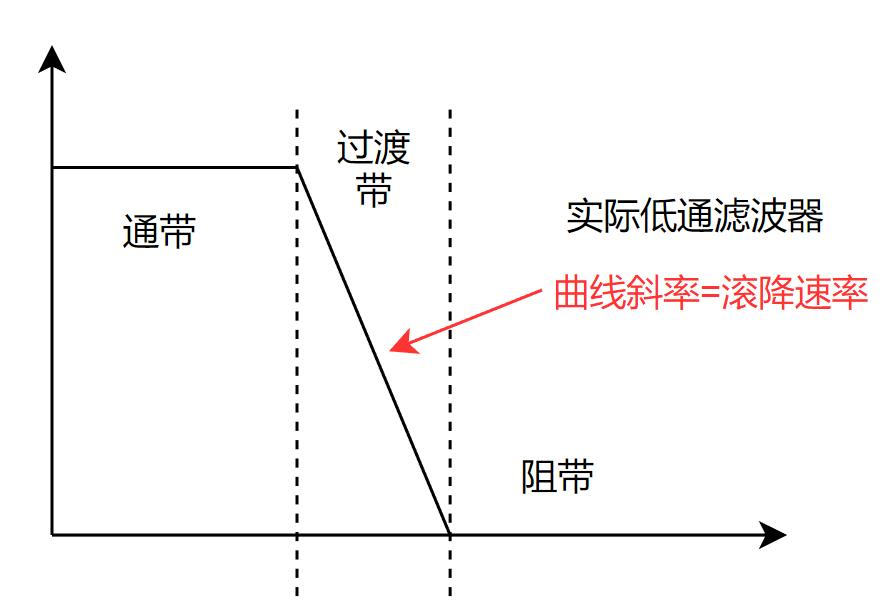• 滤波器结构前后的空间电磁场耦合,导致抑制性能下降
• 电路中的热噪声,限制了滤波器衰减的下限
• 构成滤波器的元器件的非理想参数,在抑制带形成寄生通带
• ……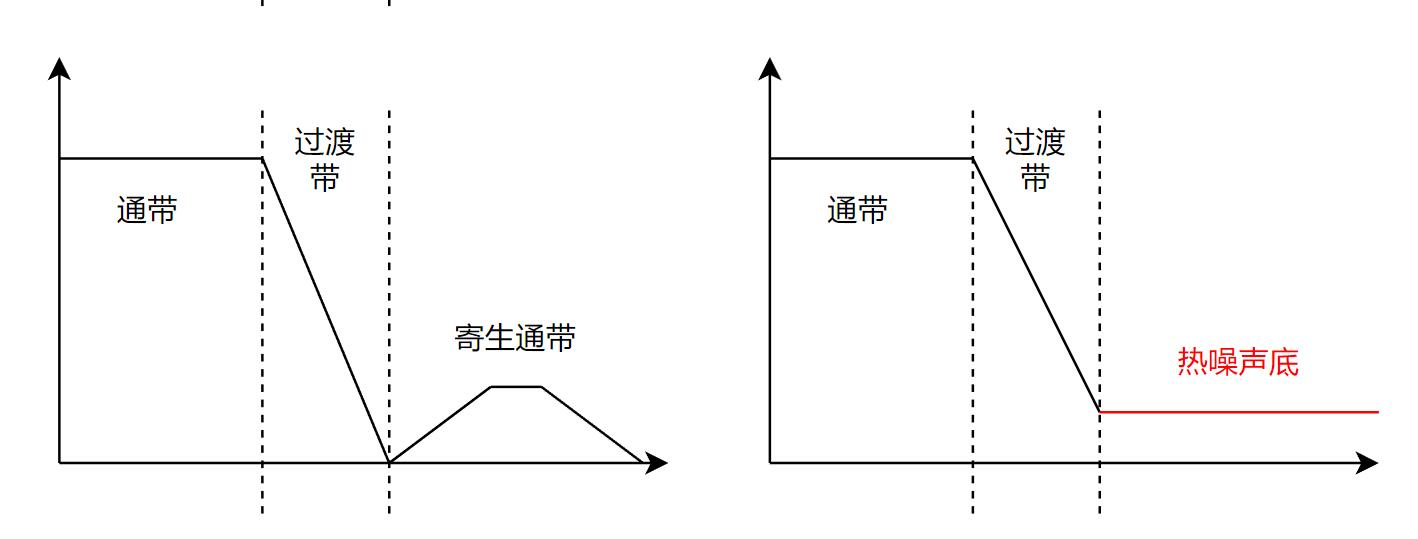(well…上面的图中，我没有标注y轴的幅度，所以这些图仅供示意。)

### 2.1.1-纹波(Ripple)## 2.2-幅度特性-滚降速率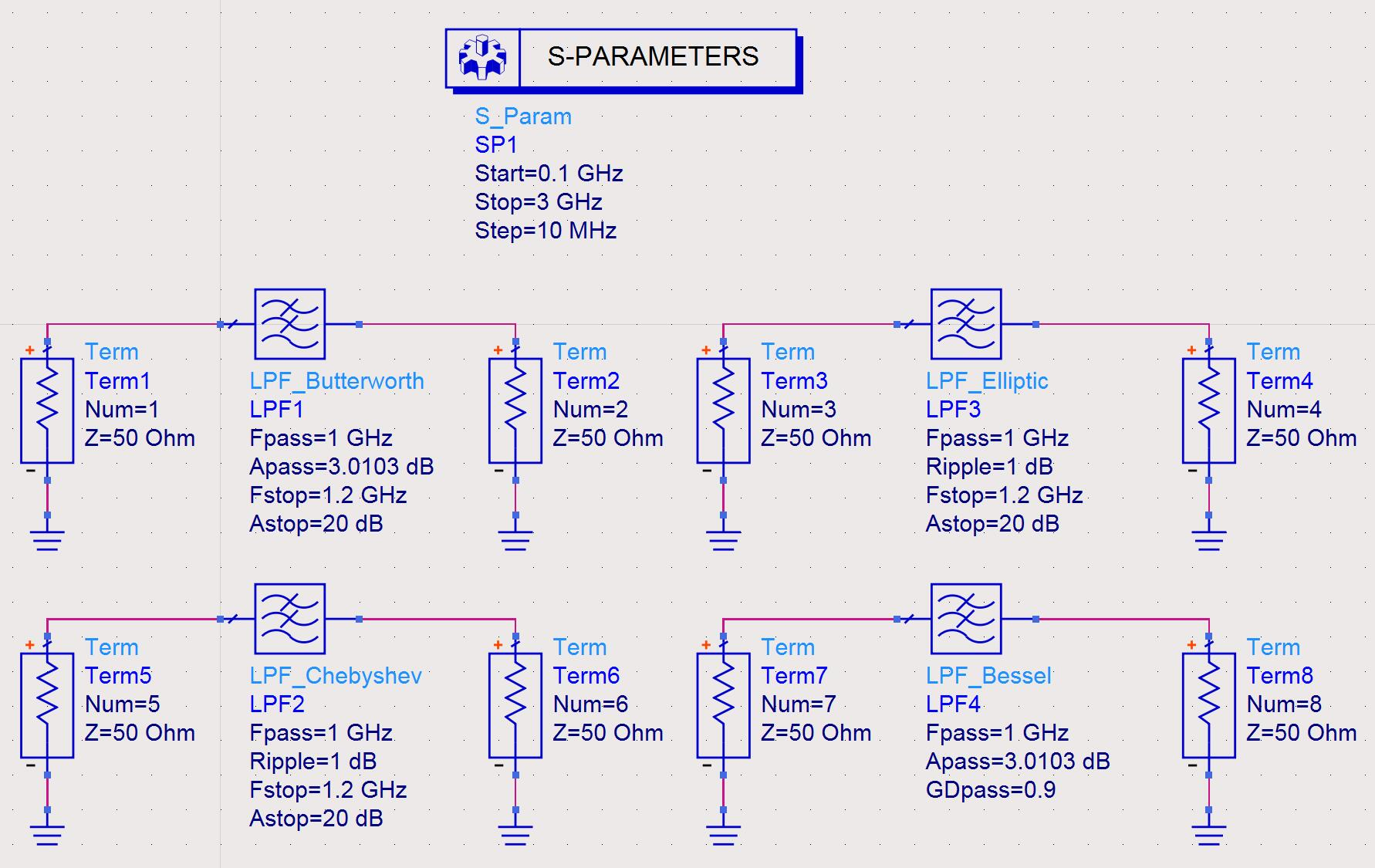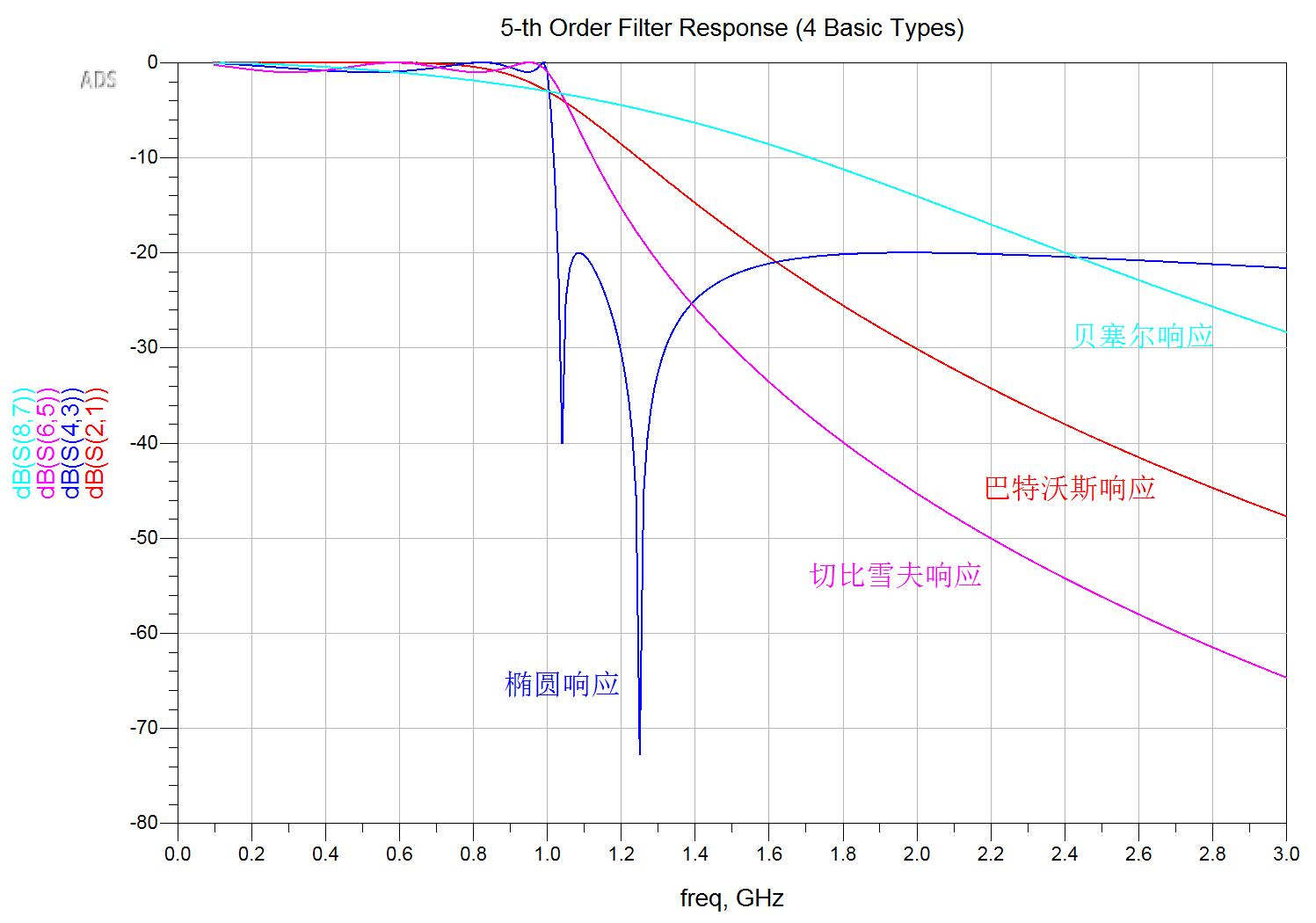### 2.2.1-估算所需滤波器的阶数

#### 2.2.1.1-一阶RC系统

H(s) = {RCs \over 1+RCs}

|H(\jmath\omega)| = {1 \over \sqrt{1+(\omega RC)^2}}

|A|^2={1\over 1+({\omega \over \omega_c})^2} = {1\over 1+\omega^2}

Insertion \ Loss(IL) = 10\log(1+\omega^2) \ (dB)

Insertion \ Loss(IL) = 10\log(\omega^2)=20\log(\omega) \ (dB)

\Delta L = 20\log ({\omega_2 \over \omega_1}) \ dB/interval_{2,1}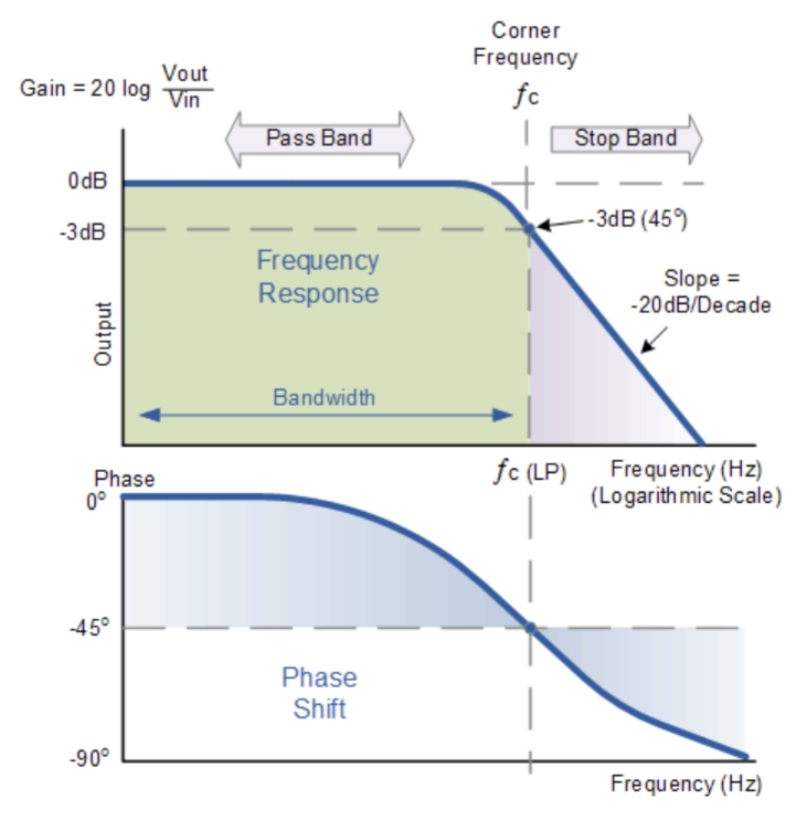### 2.2.2-N阶低通滤波器滚降计算

N阶滤波器可以由多节单阶滤波器级联而成，如果各阶滤波器前后输入输出匹配，在理想情况下N阶滤波器的滚降速率为：

\Delta L = N\cdot 20dB \ per \ decade(10倍频程) \\
\Delta L = N\cdot 6dB \ per \ octave(2倍频程)### 2.2.3-更通用的估算公式

\Delta L = N \cdot 20\log({f_2\over f_1}) \ dB/interval_{2,1}

f_0 = \sqrt{(10GHz-1GHz/2)(10GHz+1GHz/2)} = 9.9875GHz

\Delta L = N \cdot 20\log({|f_1-f_0| \over f_{BW}/2}) \ dB/interval_{1,0}

\Delta L_{10.8GHz} = 7 \cdot 20 \log({ 10.8GHz-9.9875GHz \over 1GHz/2 }) \ dB = 29.52dB \\
\Delta L_{11GHz} = 7 \cdot 20 \log({ 11GHz-9.9875GHz \over 1GHz/2 }) \ dB = 42.90dB \\
\Delta L_{13GHz} = 7 \cdot 20 \log({ 13GHz-9.9875GHz \over 1GHz/2 }) \ dB = 109.19dB \\
\Delta L_{7GHz} = 7 \cdot 20 \log({ |7GHz-9.9875GHz| \over 1GHz/2 }) \ dB = 108.69dB \\

## 2.3-相位特性-群延迟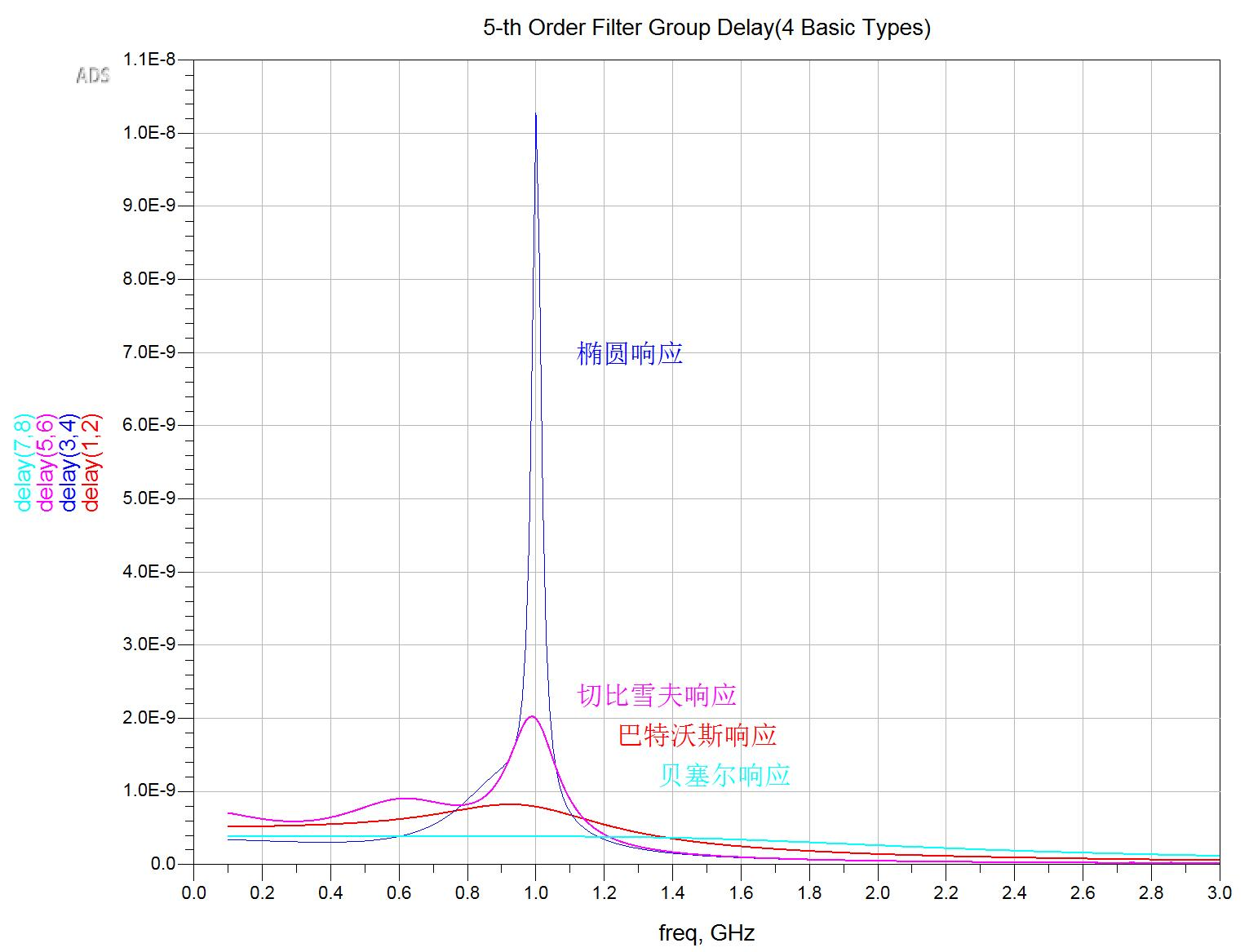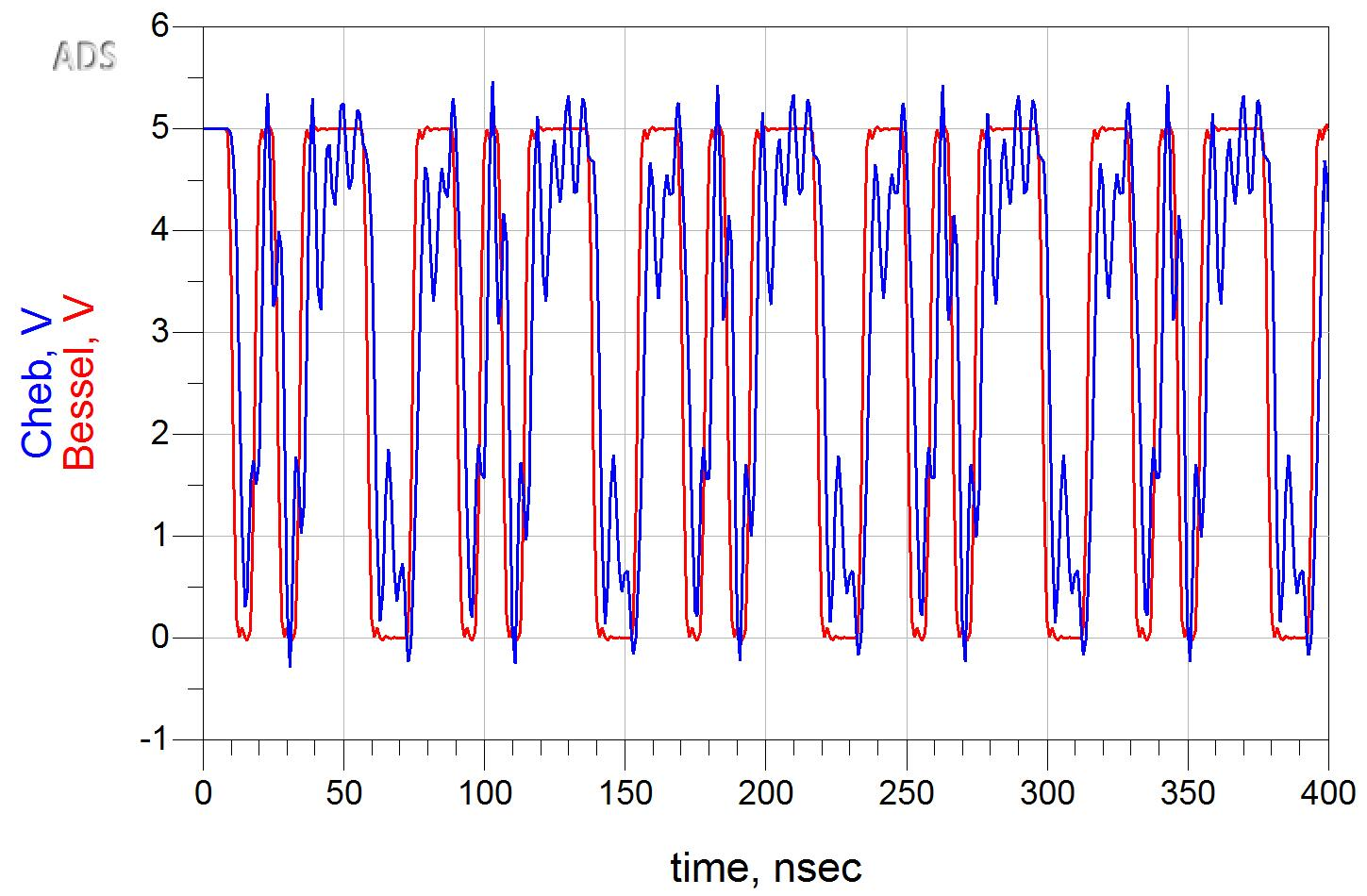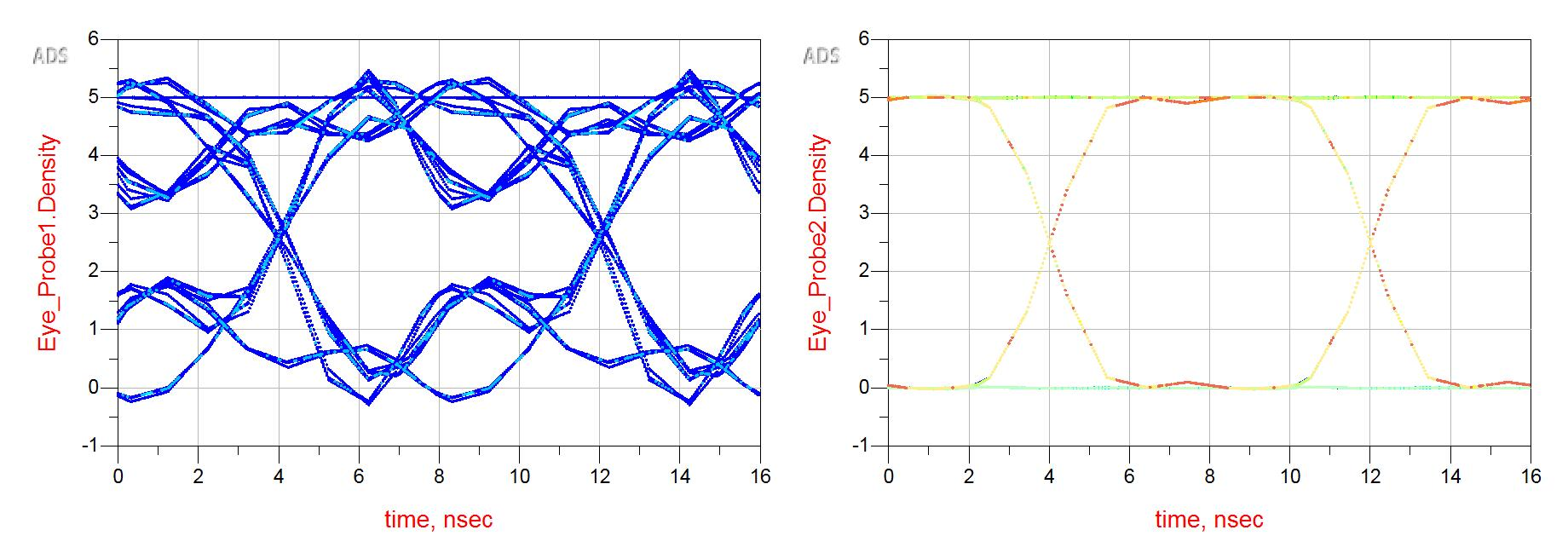• 从集总到分布
• 网络综合
• 高阶魔法—微波滤波器